Related Articles
Apply Operations To Groups In Pandas
• Last Updated : 17 Dec, 2020

Prerequisites:  Pandas

Pandas is a Python library for data analysis and data manipulation. Often data analysis requires data to be broken into groups to perform various operations on these groups. The GroupBy function in Pandas employs the split-apply-combine strategy meaning it performs a combination of — splitting an object, applying functions to the object and combining the results. In this article, we will use the groupby() function to perform various operations on grouped data.

### Aggregation

Aggregation involves creating statistical summaries of data with methods such as mean, median, mode, min (minimum), max (maximum), std (standard deviation), var (variance), sum, count, etc. To perform the aggregation operation on groups:

• Import module
• Create a GroupBy object which groups data along a key or multiple keys
• Apply a statistical operation.

Example 1: Calculate the mean salaries and age of male and female groups. It gives the mean of numeric columns and adds a prefix to the column names.

## Python3

 `# Import required libraries``import` `pandas as pd``import` `numpy as np`` ` `# Create a sample dataframe``df ``=` `pd.DataFrame({``"dept"``: np.random.choice([``"IT"``, ``"HR"``, ``"Sales"``, ``"Production"``], size``=``50``),``                   ``"gender"``: np.random.choice([``"F"``, ``"M"``], size``=``50``),``                   ``"age"``: np.random.randint(``22``, ``60``, size``=``50``),``                   ``"salary"``: np.random.randint(``20000``, ``90000``, size``=``50``)})``df.index.name ``=` `"emp_id"`` ` `# Calculate mean data of gender groups``df.groupby(``'gender'``).mean().add_prefix(``'mean_'``)`

Output: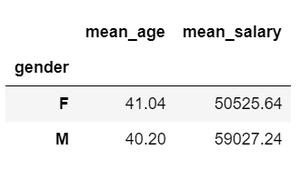Example 2: Performing multiple aggregate operations using the aggregate function (DataFrameGroupBy.agg) which accepts a string, function or a list of functions.

## Python3

 `# Import required libraries``import` `pandas as pd``import` `numpy as np`` ` `# Create a sample dataframe``df ``=` `pd.DataFrame({``"dept"``: np.random.choice([``"IT"``, ``"HR"``, ``"Sales"``, ``"Production"``], size``=``50``),``                   ``"gender"``: np.random.choice([``"F"``, ``"M"``], size``=``50``),``                   ``"age"``: np.random.randint(``22``, ``60``, size``=``50``),``                   ``"salary"``: np.random.randint(``20000``, ``90000``, size``=``50``)})``df.index.name ``=` `"emp_id"`` ` `# Calculate min, max, mean and count of salaries``# in different departments for males and females``df.groupby([``'dept'``, ``'gender'``])[``'salary'``].agg([``"min"``, ``"max"``, ``"mean"``, ``"count"``])`

Output: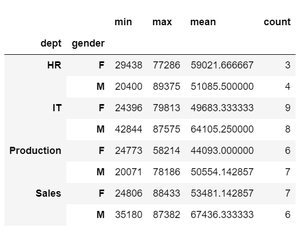Example 3: Specifying multiple columns and their corresponding aggregate operations as follows.

## Python3

 `# Import required libraries``import` `pandas as pd``import` `numpy as np`` ` `# Create a sample dataframe``df ``=` `pd.DataFrame({``"dept"``: np.random.choice([``"IT"``, ``"HR"``, ``"Sales"``, ``"Production"``], size``=``50``),``                   ``"gender"``: np.random.choice([``"F"``, ``"M"``], size``=``50``),``                   ``"age"``: np.random.randint(``22``, ``60``, size``=``50``),``                   ``"salary"``: np.random.randint(``20000``, ``90000``, size``=``50``)})``df.index.name ``=` `"emp_id"`` ` `# Calculate mean salaries and min-max age of employees``# in different departments for gender groups``df.groupby([``'dept'``, ``'gender'``]).agg({``'salary'``: ``'mean'``, ``'age'``: [``'min'``, ``'max'``]})`

Output: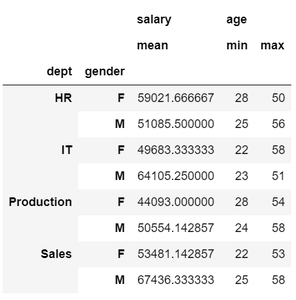Example 4: Display common statistics for any group.

## Python3

 `# Import required libraries``import` `pandas as pd``import` `numpy as np`` ` `# Create a sample dataframe``df ``=` `pd.DataFrame({``"dept"``: np.random.choice([``"IT"``, ``"HR"``, ``"Sales"``, ``"Production"``], size``=``50``),``                   ``"gender"``: np.random.choice([``"F"``, ``"M"``], size``=``50``),``                   ``"age"``: np.random.randint(``22``, ``60``, size``=``50``),``                   ``"salary"``: np.random.randint(``20000``, ``90000``, size``=``50``)})``df.index.name ``=` `"emp_id"`` ` `# Statistics of employee age grouped by departments``df[``"age"``].groupby(df[``'dept'``]).describe()`

Output: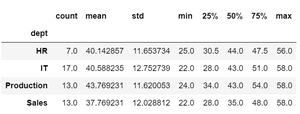Create bins or groups and apply operations

The cut method of Pandas sorts values into bin intervals creating groups or categories. Aggregation or other functions can then be performed on these groups. Implementation of this is shown below:

Example : Age is divided into age ranges and the count of observations in the sample data is calculated.

## Python3

 `# Import required libraries``import` `pandas as pd``import` `numpy as np`` ` `# Create a sample dataframe``df ``=` `pd.DataFrame({``"dept"``: np.random.choice([``"IT"``, ``"HR"``, ``"Sales"``, ``"Production"``], size``=``50``),``                   ``"gender"``: np.random.choice([``"F"``, ``"M"``], size``=``50``),``                   ``"age"``: np.random.randint(``22``, ``60``, size``=``50``),``                   ``"salary"``: np.random.randint(``20000``, ``90000``, size``=``50``)})``df.index.name ``=` `"emp_id"`` ` `# Create bin intervals``bins ``=` `[``20``, ``30``, ``45``, ``60``]`` ` `# Segregate ages into bins of age groups``df[``'categories'``] ``=` `pd.cut(df[``'age'``], bins,``                          ``labels``=``[``'Young'``, ``'Middle'``, ``'Old'``])`` ` `# Calculate number of obervations in each age category``df[``'age'``].groupby(df[``'categories'``]).count()`

Output: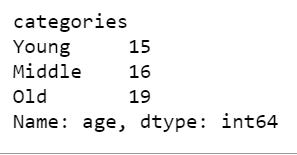### Transformation

Transformation is performing a group-specific operation where the individual values are changed while the shape of the data remains same. We use the transform() function to do so.

Example :

## Python3

 `# Import required libraries``import` `pandas as pd``import` `numpy as np`` ` `# Create a sample dataframe``df ``=` `pd.DataFrame({``"dept"``: np.random.choice([``"IT"``, ``"HR"``, ``"Sales"``, ``"Production"``], size``=``50``),``                   ``"gender"``: np.random.choice([``"F"``, ``"M"``], size``=``50``),``                   ``"age"``: np.random.randint(``22``, ``60``, size``=``50``),``                   ``"salary"``: np.random.randint(``20000``, ``90000``, size``=``50``)})``df.index.name ``=` `"emp_id"`` ` `# Calculate mean difference by transforming each salary value``df[``'mean_sal_diff'``] ``=` `df[``'salary'``].groupby(``    ``df[``'dept'``]).transform(``lambda` `x: x ``-` `x.mean())``df.head()`

Output: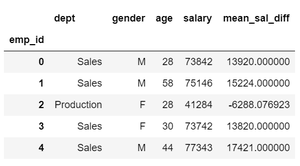Attention geek! Strengthen your foundations with the Python Programming Foundation Course and learn the basics.

To begin with, your interview preparations Enhance your Data Structures concepts with the Python DS Course. And to begin with your Machine Learning Journey, join the Machine Learning – Basic Level Course

My Personal Notes arrow_drop_up